Courses

# DC Biasing BJTs (Part - 1) Electrical Engineering (EE) Notes | EduRev

## Electrical Engineering (EE) : DC Biasing BJTs (Part - 1) Electrical Engineering (EE) Notes | EduRev

The document DC Biasing BJTs (Part - 1) Electrical Engineering (EE) Notes | EduRev is a part of Electrical Engineering (EE) category.
All you need of Electrical Engineering (EE) at this link: Electrical Engineering (EE)

DC Biasing - BJTs

Objectives To Understand :

• Concept of Operating point and stability
• Analyzing Various biasing circuits and their comparison with respect to stability

BJT – A Review

• Invented in 1948 by Bardeen, Brattain and Shockley
• Contains three adjoining, alternately doped semiconductor regions: Emitter (E), Base (B), and Collector (C)
• The middle region, base, is very thin
• Emitter is heavily doped compared to collector. So, emitter and collector are not interchangeable.

Three operating regions

• Linear – region operation: – Base – emitter junction forward biased – Base – collector junction reverse biased
• Cutoff – region operation: – Base – emitter junction reverse biased – Base – collector junction reverse biased
• Saturation – region operation: – Base – emitter junction forward biased – Base – collector junction forward biased

Three operating regions of BJT

• Cut off: VCE = VCC, IC ≌ 0
• Active or linear : VCE ≌ VCC/2 , IC ≌ IC max/2
• Saturation: VCE ≌ 0 , IC ≌ IC max

Q-Point (Static Operation Point)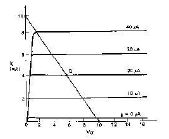• The values of the parameters IB, IC and VCE together are termed as „operating point‟ or Q ( Quiescent) point of the transistor.

Q-Point

• The intersection of the dc bias value of IB with the dc load line determines the Q-point.
• It is desirable to have the Q-point centered on the load line. Why?
• When a circuit is designed to have a centered Q-point, the amplifier is said to be midpoint biased.
• Midpoint biasing allows optimum ac operation of the amplifier.

Introduction - Biasing

The analysis or design of a transistor amplifier requires knowledge of both the dc and ac response of the system.In fact, the amplifier increases the strength of a weak signal by transferring the energy from the applied DC source to the weak input ac signal

• The analysis or design of any electronic amplifier therefore has two components:
• The dc portion and
• The ac portion During the design stage, the choice of parameters for the required dc levels will affect the ac response.

What is biasing circuit?

• Once the desired dc current and voltage levels have been identified, a network must be constructed that will establish the desired values of IB, IC and VCE, Such a network is known as biasing circuit. A biasing network has to preferably make use of one power supply to bias both the junctions of the transistor.

Purpose of the DC biasing circuit

• To turn the device “ON”
• To place it in operation in the region of its characteristic where the device operates most linearly, i.e. to set up the initial dc values of IB, IC, and VCE

Important basic relationship

• VBE = 0.7V
• IE = (b + 1) IB ≌ IC
• IC = β IB

Biasing circuits:

• Fixed – bias circuit
• Emitter bias
• Voltage divider bias
• DC bias with voltage feedback
• Miscellaneous bias

Fixed bias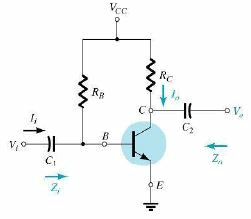• The simplest transistor dc bias configuration.
• For dc analysis, open all the capacitance.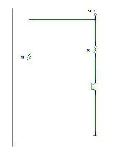DC Analysis

• Applying KVL to the input loop:
VCC = IBRB + VBE
• From the above equation, deriving for IB, we get,
IB = [VCC – VBE] / RB
• The selection of RB sets the level of base current for the operating point.
• Applying KVL for the output loop:
VCC = ICRC + VCE
• Thus,

VCE = VCC – ICRC

• In circuits where emitter is grounded, VCE = VE

VBE = VB

Design and Analysis

• Design: Given – IB, IC , VCE and VCC, or IC , VCE and β, design the values of RB, RC using the equations obtained by applying KVL to input and output loops. "
• Analysis: Given the circuit values (VCC, Rand RC), determine the values of IB, IC , VCE using the equations obtained by applying KVL to input and output loops.

Problem – Analysis

Given the fixed bias circuit with VCC = 12V, RB = 240 kΩ, RC = 2.2 kΩ and β = 75. Determine the values of operating point.

Equation for the input loop is: IB = [VCC – VBE] / RB where VBE = 0.7V, thus substituting the other given values in the equation, we get

IB = 47.08uA
IC = bIB = 3.53mA
VCE = VCC - ICR= 4.23V

•  When the transistor is biased such that IB is very high so as to make IC very high such that ICRC drop is almost VCC and VCE is almost 0, the transistor is said to be in saturation. IC sat = VCC / RC in a fixed bias circuit.

Verification

• Whenever a fixed bias circuit is analyzed, the value of ICQ obtained could be verified with the value of ICSat ( = VCC / RC) to understand whether the transistor is in active region.
• In active region,

ICQ = ( ICSat /2)

Load line analysis

A fixed bias circuit with given values of VCC, RC and RB can be analyzed ( means, determining the values of IBQ, ICQ and VCEQ) using the concept of load line also. Here the input loop KVL equation is not used for the purpose of analysis, instead, the output characteristics of the transistor used in the given circuit and output loop KVL equation are made use of.

• The method of load line analysis is as below:
1. Consider the equation VCE = VCC – ICRC This relates VCE and IC for the given IB and RC
2. Also, we know that, VCE and IC are related through output characteristics We know that the equation, VCE = VCC – ICRC represents a straight line which can be plotted on the output characteristics of the transistor.

Such line drawn as per the above equation is known as load line, the slope of which is decided by the value of RC ( the load).

Load line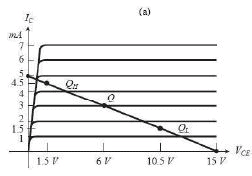• The two extreme points on the load line can be calculated and by joining which the load line can be drawn.
• To find extreme points, first, Ic is made 0 in the equation: VCE = VCC – ICRC . This gives the coordinates (VCC,0) on the x axis of the output characteristics.
• The other extreme point is on the y-axis and can be calculated by making VCE = 0 in the equation VCE = VCC – ICRC which gives IC( max) = VCC / RC thus giving the coordinates of the point as (0, VCC / RC).
• The two extreme points so obtained are joined to form the load line.
• The load line intersects the output characteristics at various points corresponding to different IBs. The actual operating point is established for the given IB

Q point variation

As IB is varied, the Q point shifts accordingly on the load line either up or down depending on IB increased or decreased respectively.
As RC is varied, the Q point shifts to left or right along the same IB line since the slope of the line varies. As RC increases, slope reduces ( slope is -1/RC) which results in shift of Q point to the left meaning no variation in IC and reduction in VCE . Thus if the output characteristics is known, the analysis of the given fixed bias circuit or designing a fixed bias circuit is possible using load line analysis as mentioned above.

Emitter Bias

• It can be shown that, including an emitter resistor in the fixed bias circuit improves the stability of Q point.
• Thus emitter bias is a biasing circuit very similar to fixed bias circuit with an emitter resistor added to it.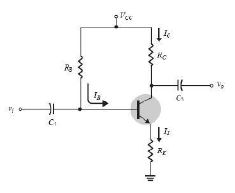Input loop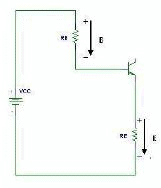• Writing KVL around the input loop we get, VCC = IBRB + VBE + IERE              (1)

We know that,
IE = (β+1)IB (2)
Substituting this in (1), we get,
VCC = IBRB + VBE + (b+1)IBRE
VCC – VBE = IB(RB + (b+1) RE)
Solving for IB:
IB = (VCC – VBE ) /[(RB + (b+1) RE)]

The expression for IB in a fixed bias circuit was, IB = (VCC – VBE ) /R,

Equivalent input loop: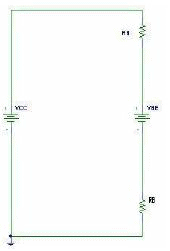• REI in the above circuit is (β +1)RE which means that, the emitter resistance that is common to both the loops appears as such a high resistance in the input loop. " Thus R= (β+1)RE ( more about this when we take up ac analysis)

Output loop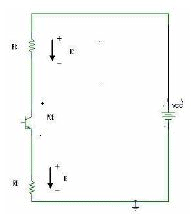Collector – emitter loop Applying KVL,

VCC = ICRC + VCE + IERE

IC is almost same as IE

Thus,
VCC = ICRC + VCE + ICRE
= IC (RC + RE) +VCE
VCE = VCC - IC (RC + RE)
Since emitter is not connected directly to ground, it is at a potential VE, given by,
VE = IERE
VC = VCE + VE OR VC = VCC – ICRC
Also, VB = VCC – IBRB OR VB = VBE + VE

Problem:

Analyze the following circuit: given b = 75, VCC = 16V, RB = 430kΩ, R= 2kΩ and RE = 1k Ω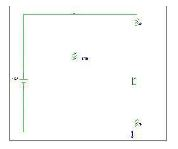Solution:

IB = (VCC – VBE ) /[(RB + (b+1) RE)]
= ( 16 – 0.7) / [ 430k + (76) 1k] = 30.24mA IC = ( 75) (30.24mA) = 2.27mA VCE = VCC - IC (RC + RE) = 9.19V
V= VCC – ICRC = 11.46V
VE = VC – VCE = 2.27V
VB = VBE + VE = 2.97V
VBC = VB – VC = 2.97 – 11.46 = - 8.49V

Offer running on EduRev: Apply code STAYHOME200 to get INR 200 off on our premium plan EduRev Infinity!

,

,

,

,

,

,

,

,

,

,

,

,

,

,

,

,

,

,

,

,

,

;